#### 期刊菜单

Research on Traffic Safety Risk Assessment and Temporary Safety Protection System in Expressway Reconstruction and Expansion Operation Area
DOI: 10.12677/HJCE.2022.118102, PDF , HTML, XML, 下载: 145  浏览: 225  科研立项经费支持

Abstract: Aiming at the inadequacy of temporary traffic safety protection in the expressway reconstruction and expansion operation area, the engineering characteristics and traffic operation characteristics of the expressway reconstruction and expansion operation area are systematically analyzed. The driving safety risk index system of the reconstruction and expansion operation area is constructed, the grey clustering evaluation method is used to analyze the driving safety risk of the reconstruction and expansion operation area, the six-level risk evaluation system is established, and the suggestions for setting temporary traffic safety protection system are put forward, which can provide a reference for similar reconstruction and expansion projects.

1. 引言

2. 作业区行车安全要素及指标构建

2.1. 作业区道路条件

2.2. 作业区时长 

2.3. 改扩建作业区交通组织

1) 交通量状况

2) 改扩建作业区主动引导体系Table 1. Influencing traffic safety risk index group in the reconstruction and expansion operation area

3. 指标变量及权重

1) 圆曲线变量X1

X1为集计变量，标识评价路段平均百米长度的偏角值，按下式计算。

${X}_{1}=\sum \left(W{H}_{i}×DE{G}_{i}\right)$

L——评价路段的长度；

Ri——第i个圆曲线半径；

WHi——第i个圆曲线位于评价路段内的比重， $WH={l}_{i}/L$

DEGi——圆曲线i每百米长度的曲线偏角， $DEG=18000/\text{π}/R$

2) 纵坡变量X2

X2为集计变量，表示评价路段加权平均纵坡值，按下式计算。

${X}_{2}=\frac{\sum \left({G}_{i}×{l}_{i}\right)}{L}$

li——坡段长度；

L——评价路段的总长度。

3) 小时交通量变量X3

4) 大型车比重变量X4

${X}_{4}=\frac{\sum Truc{k}_{i}}{ADT}×100%$

5) 作业区施工时长变量X5

X5在通常是指一个地点设置作业区，实施作业以及拆除作业区的时间总和。

6) 作业区长度变量X6

X6通常是指警告区、上游过渡段、缓冲段、施工区、下游过渡段的总和。

7) 封闭车道宽度X7

8) 路侧净区宽度满足率X8

${X}_{8}=\frac{CZ}{W}×100%$

π = αW，α——折减系数，取值在0.6~0.8之间；

W——依据评价路段的边坡比率、交通量和设计速度,参考美国《路侧设计指南》  关于路侧净区设置之规定，得到建议净区宽度的下限值。

9) 作业区限速变量X9

10) 作业区诱导设施变量X10Table 2. Weight table of evaluation index variables of safety risk level in operation area

4. 灰类函数白化函数的构造

1) 对于路侧安全等级为1级的情况，用下限白化权函数

${f}_{j}^{1}\left(x\right)=\left\{\begin{array}{l}0\text{​}\text{​}\text{​}\text{\hspace{0.17em}}\text{\hspace{0.17em}}\text{\hspace{0.17em}}\text{\hspace{0.17em}}\text{\hspace{0.17em}}\text{\hspace{0.17em}}\text{\hspace{0.17em}}\text{\hspace{0.17em}}\text{\hspace{0.17em}}\text{\hspace{0.17em}}\text{\hspace{0.17em}}\text{\hspace{0.17em}}\text{\hspace{0.17em}}x>{A}_{2}\\ \frac{{A}_{2}-x}{{A}_{2}-{A}_{1}}\text{\hspace{0.17em}}\text{ }\text{ }\text{ }\text{ }\text{\hspace{0.17em}}\text{\hspace{0.17em}}x\in \left[{A}_{1},{A}_{2}\right]\\ 1\text{\hspace{0.17em}}\text{\hspace{0.17em}}\text{\hspace{0.17em}}\text{\hspace{0.17em}}\text{\hspace{0.17em}}\text{\hspace{0.17em}}\text{\hspace{0.17em}}\text{\hspace{0.17em}}\text{\hspace{0.17em}}\text{\hspace{0.17em}}\text{\hspace{0.17em}}\text{\hspace{0.17em}}\text{\hspace{0.17em}}x<{A}_{1}\end{array}$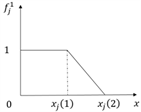2) 对于路侧安全等级为2级的情况，用适中测度的白化权函数

${f}_{j}^{2}\left(x\right)=\left\{\begin{array}{l}0\text{​}\text{​}\text{​}\text{\hspace{0.17em}}\text{\hspace{0.17em}}\text{\hspace{0.17em}}\text{\hspace{0.17em}}\text{\hspace{0.17em}}\text{\hspace{0.17em}}\text{\hspace{0.17em}}\text{\hspace{0.17em}}\text{\hspace{0.17em}}\text{\hspace{0.17em}}\text{\hspace{0.17em}}\text{\hspace{0.17em}}\text{\hspace{0.17em}}x\notin \left[{A}_{1},{A}_{3}\right]\\ \frac{x-{A}_{1}}{{\lambda }_{j}^{2}-{A}_{1}}\text{\hspace{0.17em}}\text{ }\text{ }\text{ }\text{ }\text{\hspace{0.17em}}\text{\hspace{0.17em}}x\in \left[{A}_{1},{\lambda }_{j}^{2}\right]\\ \frac{{A}_{3}-x}{{A}_{3}-{\lambda }_{j}^{2}}\text{\hspace{0.17em}}\text{ }\text{ }\text{ }\text{ }\text{\hspace{0.17em}}\text{\hspace{0.17em}}x\in \left[{\lambda }_{j}^{2},{A}_{3}\right]\end{array}$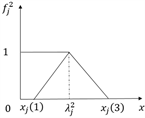3) 对于路侧安全等级为3级的情况，用适中测度的白化权函数

${f}_{j}^{3}\left(x\right)=\left\{\begin{array}{l}0\text{​}\text{​}\text{​}\text{\hspace{0.17em}}\text{\hspace{0.17em}}\text{\hspace{0.17em}}\text{\hspace{0.17em}}\text{\hspace{0.17em}}\text{\hspace{0.17em}}\text{\hspace{0.17em}}\text{\hspace{0.17em}}\text{\hspace{0.17em}}\text{\hspace{0.17em}}\text{\hspace{0.17em}}\text{\hspace{0.17em}}\text{\hspace{0.17em}}x\notin \left[{A}_{2},{A}_{4}\right]\\ \frac{x-{A}_{2}}{{\lambda }_{j}^{3}-{A}_{2}}\text{\hspace{0.17em}}\text{ }\text{ }\text{ }\text{ }\text{\hspace{0.17em}}\text{\hspace{0.17em}}x\in \left[{A}_{2},{\lambda }_{j}^{2}\right]\\ \frac{{A}_{4}-x}{{A}_{4}-{\lambda }_{j}^{3}}\text{\hspace{0.17em}}\text{ }\text{ }\text{ }\text{ }\text{\hspace{0.17em}}\text{\hspace{0.17em}}x\in \left[{\lambda }_{j}^{3},{A}_{4}\right]\end{array}$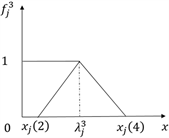4) 对于路侧安全等级为4级的情况，用适中测度的白化权函数

${f}_{j}^{4}\left(x\right)=\left\{\begin{array}{l}0\text{​}\text{​}\text{​}\text{\hspace{0.17em}}\text{\hspace{0.17em}}\text{\hspace{0.17em}}\text{\hspace{0.17em}}\text{\hspace{0.17em}}\text{\hspace{0.17em}}\text{\hspace{0.17em}}\text{\hspace{0.17em}}\text{\hspace{0.17em}}\text{\hspace{0.17em}}\text{\hspace{0.17em}}\text{\hspace{0.17em}}\text{\hspace{0.17em}}x\notin \left[{A}_{3},{A}_{5}\right]\\ \frac{x-{A}_{3}}{{\lambda }_{j}^{4}-{A}_{3}}\text{\hspace{0.17em}}\text{ }\text{ }\text{ }\text{ }\text{\hspace{0.17em}}\text{\hspace{0.17em}}x\in \left[{A}_{3},{\lambda }_{j}^{4}\right]\\ \frac{{A}_{5}-x}{{A}_{5}-{\lambda }_{j}^{4}}\text{\hspace{0.17em}}\text{ }\text{ }\text{ }\text{ }\text{\hspace{0.17em}}\text{\hspace{0.17em}}x\in \left[{\lambda }_{j}^{4},{A}_{5}\right]\end{array}$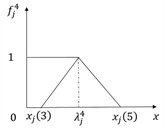5) 对于路侧安全等级为5级的情况，用适中测度的白化权函数

${f}_{j}^{5}\left(x\right)=\left\{\begin{array}{l}0\text{​}\text{​}\text{​}\text{\hspace{0.17em}}\text{\hspace{0.17em}}\text{\hspace{0.17em}}\text{\hspace{0.17em}}\text{\hspace{0.17em}}\text{\hspace{0.17em}}\text{\hspace{0.17em}}\text{\hspace{0.17em}}\text{\hspace{0.17em}}\text{\hspace{0.17em}}\text{\hspace{0.17em}}\text{\hspace{0.17em}}\text{\hspace{0.17em}}x\notin \left[{A}_{4},{A}_{6}\right]\\ \frac{x-{A}_{4}}{{\lambda }_{j}^{5}-{A}_{4}}\text{\hspace{0.17em}}\text{ }\text{ }\text{ }\text{ }\text{\hspace{0.17em}}\text{\hspace{0.17em}}x\in \left[{A}_{4},{\lambda }_{j}^{5}\right]\\ \frac{{A}_{6}-x}{{A}_{6}-{\lambda }_{j}^{5}}\text{\hspace{0.17em}}\text{ }\text{ }\text{ }\text{ }\text{\hspace{0.17em}}\text{\hspace{0.17em}}x\in \left[{\lambda }_{j}^{5},{A}_{6}\right]\end{array}$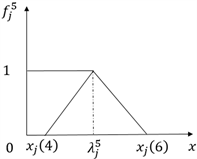6) 对于路侧安全等级为6级的情况，用下限白化权函数

${f}_{j}^{1}\left(x\right)=\left\{\begin{array}{l}0\text{​}\text{​}\text{​}\text{\hspace{0.17em}}\text{\hspace{0.17em}}\text{\hspace{0.17em}}\text{\hspace{0.17em}}\text{\hspace{0.17em}}\text{\hspace{0.17em}}\text{\hspace{0.17em}}\text{\hspace{0.17em}}\text{\hspace{0.17em}}\text{\hspace{0.17em}}\text{\hspace{0.17em}}\text{\hspace{0.17em}}\text{\hspace{0.17em}}x<{A}_{5}\\ \frac{{A}_{2}-x}{{A}_{2}-{A}_{1}}\text{\hspace{0.17em}}\text{ }\text{ }\text{ }\text{ }\text{\hspace{0.17em}}\text{\hspace{0.17em}}x\in \left[{A}_{5},{A}_{6}\right]\\ 1\text{\hspace{0.17em}}\text{\hspace{0.17em}}\text{\hspace{0.17em}}\text{\hspace{0.17em}}\text{\hspace{0.17em}}\text{\hspace{0.17em}}\text{\hspace{0.17em}}\text{\hspace{0.17em}}\text{\hspace{0.17em}}\text{\hspace{0.17em}}\text{\hspace{0.17em}}\text{\hspace{0.17em}}\text{\hspace{0.17em}}x>{A}_{6}\end{array}$Table 3. Rank value of the indicator system variable

5. 典型高速公路改扩建作业区行车安全风险评价

①收集线形、交通量、事故和路侧特征数据。

②将被评价路段划分成n个评价路段单元。

③计算第i个评价路段单元的变量值 ${X}_{i},j=1,2,\cdots ,10$

④计算第i个评价路段单元的4个级别白化权函数值 ${f}_{j}^{k}\left({X}_{ij}\right)$$k=1,2,3,\cdots ,5,6$

⑤计算第i个评价路段单元的灰色聚类系数 ${\sigma }_{i}^{k}$${\sigma }_{i}^{k}=\sum {f}_{j}^{k}\left({X}_{ij}\right)\ast {\eta }_{j}$

⑥确定第i个评价路段单元的路侧安全等级，若 ${\sigma }_{i}^{k}=\mathrm{max}\left\{{\sigma }_{i}^{k}\right\}$，则路段i的路侧安全等级为k*

⑦重复步骤③~⑥，直至确定所有待评价路段单元的改扩建作业区安全风险等级。

1) 封闭内侧车道短期施工，见表4Table 4. Summary table of safety risk level assessment in operation area

${\sigma }_{i}^{k}=\mathrm{max}\left\{{\sigma }_{i}^{k}\right\}=\mathrm{max}\left\{0.3043,0.3748,0.2941,0.0557,0,0\right\}=0.3748$，可知该路段路侧安全等级为2级。

2) 封闭内侧车道长期施工，见表5Table 5. Summary table of safety risk level assessment in operation area

${\sigma }_{i}^{k}=\mathrm{max}\left\{{\sigma }_{i}^{k}\right\}=\mathrm{max}\left\{0.3043,0.2791,0..3060,0.0660,0,0\right\}=0.3060$，可知该路段路侧安全等级为3级。

3) 交通转换路段，见表6Table 6. Summary table of safety risk level assessment in operation area

${\sigma }_{i}^{k}=\mathrm{max}\left\{{\sigma }_{i}^{k}\right\}=\mathrm{max}\left\{0.2342,0.1536,0.0410,0.2522,0.1243,0.1197\right\}=0.2522$，可知该路段路侧安全等级为4级。

4) 单幅双向通行路段，见表7Table 7. Summary table of safety risk level assessment in operation area

${\sigma }_{i}^{k}=\mathrm{max}\left\{{\sigma }_{i}^{k}\right\}=\mathrm{max}\left\{0.3043,0.1424,0.0325,0,0.3526,0.3486\right\}=0.3525$，可知该路段路侧安全等级为5级。

5) 当路段交通组织较小，作业区施工时长为短时时，易知其路侧安全等级为1级。当施工路段为桥梁，路侧为水源、公跨铁，高边坡、保护区域等，易知路侧安全等级为6级。

6. 高速公路改扩建作业区行车安全风险等级及应对Table 8. Suggestions on setting temporary safety protection classification based on driving safety risk level in operation area

7. 小结

  程家富. 高速公路改扩建工程交通安全隐患及预防措施研究[J]. 筑路机械与施工机械化, 2012, 29(6): 59-62.  中华人民共和国国家质量监督检验检疫总局, 中国国家标准化管理委员会. GB5768.4-2017. 道路交通标志和标线第4部分作业区[S]. 北京: 中国标准出版社, 2017.  王松根. JTG H30-2015. 公路养护安全作业规程[S]. 北京: 人民交通出版社, 2015.  戴坤阳, 苏建安, 毛红日. 多因素耦合作用影响的高速公路改扩建工程施工安全评价研究[J]. 项目管理技术, 2018, 16(2): 82-86.  Akintoye, A.S. and Macleod, M.J. (1997) Risk Analysis and Management in Construction. International Journal of Project Management, 15, 31-38. https://doi.org/10.1016/S0263-7863(96)00035-X